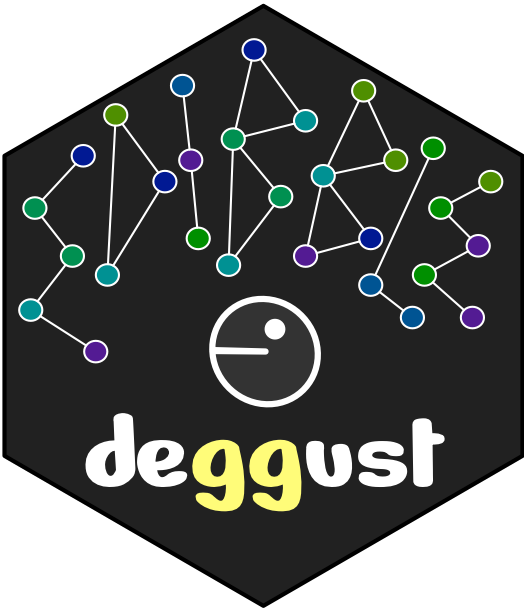## Automated visualisation of experimental designs

👩🏻‍💻

• emi.tanaka@monash.edu
• @statsgen
• github.com/emitanaka
• emitanaka.org

29th Novemebr 2022 Australasian Applied Statistics Conference 2022

## edibble R package 📦🎯 To construct an experimental design table (or tibble)

An object oriented programming system to construct an experimental design by encapsulating the experimental structure and context in a cognitive approach.

• Combine words (functions) = Unlimited sentences (designs)
• Health check

## Demonstration for edibble 📦

Steinauer et al. (2015) Plant diversity effects on soil microbial functions and enzymes are stronger than warming in a grassland experiment. Ecology. 96 (1) 99-112

library(edibble)
design("Steinauer et al. 2015") 

## Demonstration for edibble 📦

Steinauer et al. (2015) Plant diversity effects on soil microbial functions and enzymes are stronger than warming in a grassland experiment. Ecology. 96 (1) 99-112

library(edibble)
design("Steinauer et al. 2015") %>%
set_units(plot = 27,
subplot = nested_in(plot, 3))

## Demonstration for edibble 📦

Steinauer et al. (2015) Plant diversity effects on soil microbial functions and enzymes are stronger than warming in a grassland experiment. Ecology. 96 (1) 99-112

library(edibble)
design("Steinauer et al. 2015") %>%
set_units(plot = 27,
subplot = nested_in(plot, 3)) %>%
set_trts(plant_diversity = c(1, 4, 16),
temperature = c("0°C", "1.5°C", "3°C")) 

## Demonstration for edibble 📦

Steinauer et al. (2015) Plant diversity effects on soil microbial functions and enzymes are stronger than warming in a grassland experiment. Ecology. 96 (1) 99-112

library(edibble)
design("Steinauer et al. 2015") %>%
set_units(plot = 27,
subplot = nested_in(plot, 3)) %>%
set_trts(plant_diversity = c(1, 4, 16),
temperature = c("0°C", "1.5°C", "3°C")) %>%
allot_trts(plant_diversity ~ plot,
temperature ~ subplot)

## Demonstration for edibble 📦

Steinauer et al. (2015) Plant diversity effects on soil microbial functions and enzymes are stronger than warming in a grassland experiment. Ecology. 96 (1) 99-112

library(edibble)
design("Steinauer et al. 2015") %>%
set_units(plot = 27,
subplot = nested_in(plot, 3)) %>%
set_trts(plant_diversity = c(1, 4, 16),
temperature = c("0°C", "1.5°C", "3°C")) %>%
allot_trts(plant_diversity ~ plot,
temperature ~ subplot) %>%
assign_trts("random")

## Demonstration for edibble 📦

Steinauer et al. (2015) Plant diversity effects on soil microbial functions and enzymes are stronger than warming in a grassland experiment. Ecology. 96 (1) 99-112

library(edibble)
design("Steinauer et al. 2015") %>%
set_units(plot = 27,
subplot = nested_in(plot, 3)) %>%
set_trts(plant_diversity = c(1, 4, 16),
temperature = c("0°C", "1.5°C", "3°C")) %>%
allot_trts(plant_diversity ~ plot,
temperature ~ subplot) %>%
assign_trts("random") %>%
serve_table()

## Demonstration for edibble 📦

Steinauer et al. (2015) Plant diversity effects on soil microbial functions and enzymes are stronger than warming in a grassland experiment. Ecology. 96 (1) 99-112

library(edibble)
design("Steinauer et al. 2015") %>%
set_units(plot = 27,
subplot = nested_in(plot, 3)) %>%
set_trts(plant_diversity = c(1, 4, 16),
temperature = c("0°C", "1.5°C", "3°C")) %>%
allot_trts(plant_diversity ~ plot,
temperature ~ subplot) %>%
assign_trts("random") %>%
serve_table() -> des

Let’s save this edibble design object as des.

## Internal structure of an edibble design object

Factor graph

plot(des) # OR plot(des, which = "factors") OR plot_fct_graph(des)

## Internal structure of an edibble design object

Level graph

plot(des, which = "levels") # OR plot_lvl_graph(des)

## deggust R package 📦🎯 The design of experiment as a ggplot object

• degust (verb) to taste or savor carefully or appreciatively.
• deggust (package) to visualise edibble design objects appreciatively.
• An edibble design object contains encoding with experimental structure already.
• The deggust package aims to leverage this to quickly view this.

## Easy-and-quick visualisation

library(deggust)
autoplot(des)

Yup that’s all!

## Cutomise visualisation

library(deggust)
autoplot(des, aspect_ratio = 3/2)

## Cutomise visualisation with ggplot2

library(deggust)
autoplot(des, aspect_ratio = 3/2) +
theme(
legend.position = "bottom",
text = element_text(size = 18),
plot.background =
element_rect(color = "black",
linetype = "dashed",
fill = "wheat"),
plot.margin = margin(20, 20, 20, 20),
plot.subtitle =
element_text(margin = margin(0, 0, 10, 0))) +

scale_fill_brewer(palette = "Reds")

Leveraging the power of established graphical system.

# Recipe designs

## Completely randomised designs

crd <- design("CRD") %>%
set_units(unit = 30) %>%
set_trts(trt = 5) %>%
allot_trts(trt ~ unit) %>%
assign_trts("random", seed = 2022) %>%
serve_table()

autoplot(crd)

## Randomised complete block designs

rcbd <- design("RCBD") %>%
set_units(block = 10,
unit = nested_in(block, 6)) %>%
set_trts(trt = 6) %>%
allot_trts(trt ~ unit) %>%
assign_trts("random", seed = 2022) %>%
serve_table()

autoplot(rcbd)

## Modified block design

mbd <- design("Modified Block Design") %>%
set_units(block = 10,
unit = nested_in(block,
1 ~ 3,
2 ~ 4,
3 ~ 5,
. ~ 6)) %>%
set_trts(trt = 6) %>%
allot_trts(trt ~ unit) %>%
assign_trts("random", seed = 2022) %>%
serve_table()

autoplot(mbd)

## Graeco-Latin square design

glsd <- design("GLSD") %>%
set_units(row = 9,
col = 9,
unit = crossed_by(row, col)) %>%
set_trts(trt1 = 9,
trt2 = 9) %>%
allot_trts(trt1 ~ unit,
trt2 ~ unit) %>%
assign_trts("random", seed = 2022) %>%
serve_table()

autoplot(glsd)

## Hyper-Graeco-Latin Square Design

hglsd <- design("HGLSD") %>%
set_units(block1 = 4,
block2 = 4,
block3 = 4,
block4 = 4,
unit = crossed_by(block1,
block2,
block3,
block4)) %>%
set_trts(trt = 4) %>%
allot_trts(trt ~ unit) %>%
assign_trts("random", seed = 2022) %>%
serve_table()

autoplot(hglsd)

edibble R packageTo construct experimental design objects.

On CRAN On GitHub github.com/emitanaka/edibble

deggust R packageTo visualise edibble designs.

On GitHub
github.com/emitanaka/deggust

Slides at emitanaka.org/slides/AASC2022/